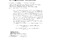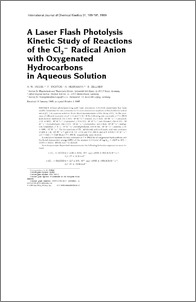### A laser flash photolysis study of reactions of the Cl2- radical anion with oxygenated hydrocarbons in aqueous solution

Contact
hwjacobi [ at ] awi-bremerhaven.de

Abstract

A laser photolysis-long path laser absorption (LP-LPLA) experiment has been used to determine the rate constants for H-atom abstraction reactions of thedichloride radical anion (Cl2-) in aqueous solution. From direct measurements of the decay of Cl2- in the presence of different reactants at pH = 4 and I = 0.1 M thefollowing rate constants at T = 298 K were derived: methanol, (5.1 ± 0.3)·104 M-1 s-1; ethanol, (1.2 ± 0.2)·105 M-1 s-1; 1-propanol, (1.01 ± 0.07)·105 M-1 s-1;2-propanol, (1.9 ± 0.3)·105 M-1 s-1; tert.-butanol, (2.6 ± 0.5)·104 M-1 s-1; formaldehyde, (3.6 ± 0.5)·104 M-1 s-1; diethylether, (4.0 ± 0.2)·105 M-1 s-1;methyl-tert.-butylether, (7 ± 1)·104 M-1 s-1; tetrahydrofuran, (4.8 ± 0.6)·105 M-1 s-1; acetone, (1.41 ± 0.09)·103 M-1 s-1. For the reactions of Cl2- with formic acidand acetic acid rate constants of (8.0 ± 1.4)·104 M-1 s-1 (pH = 0, I = 1.1 M and T = 298 K) and (1.5 ± 0.8) · 103 M-1 s-1 (pH = 0.42, I = 0.48 M and T = 298K), respectively, were derived.A correlation between the rate constants at T = 298 K for all oxygenated hydrocarbons and the bond dissociation energy (BDE) of the weakest C-H-bond of logk2nd = (32.9 ± 8.9) - (0.073 ± 0.022)·BDE/kJ mol-1 is derived.From temperature-dependent measurements the following Arrhenius expressions were derived:k (Cl2- + HCOOH) = (2.00 ± 0.05)·1010·exp(-(4500 ± 200) K/T) M-1 s-1,Ea = (37 ± 2) kJ mol-1k (Cl2- + CH3COOH) = (2.7 ± 0.5)·1010·exp(-(4900 ± 1300) K/T) M-1 s-1,Ea = (41 ± 11) kJ mol-1k (Cl2- + CH3OH) = (5.1 ± 0.9)·1012·exp(-(5500 ± 1500) K/T) M-1 s-1,Ea = (46 ± 13) kJ mol-1k (Cl2- + CH2(OH)2) = (7.9 ± 0.7)·1010·exp(-(4400 ± 700) K/T) M-1 s-1,Ea = (36 ± 5) kJ mol-1Finally, in measurements at different ionic strengths (I) a decrease of the rate constant with increasing I has been observed in the reactions of Cl2- with methanol andhydrated formaldehyde.

Item Type
Article
Authors
Divisions
Programs
Publication Status
Published
Eprint ID
1502
Cite as
Jacobi, H. W. , Wicktor, F. , Herrmann, H. and Zellner, R. (1999): A laser flash photolysis study of reactions of the Cl2- radical anion with oxygenated hydrocarbons in aqueous solution , International journal of chemical kinetics, 31 , pp. 169-181 .Preview
PDF (Fulltext)
Jac1999a.pdf